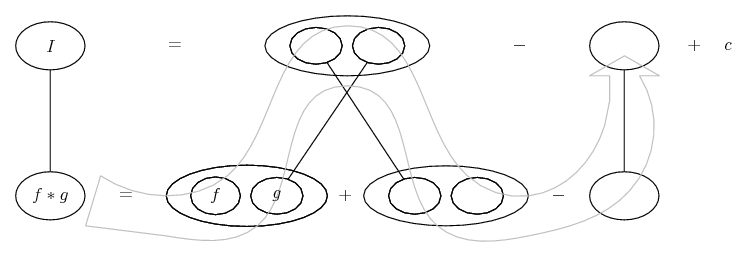# Thread: Integration of parts

1. ## Integration of parts

Stuck on a question using integration of parts.
integral sign x^2ln4x dx
u=ln4x v=1/3x^3
du= dv=x^2

S u dv = uv - S v du
ln4x( )-S1/2x^3( )
I don't know how to find du above? Is it 1/x(4x)??

Any help would be great, thanks.2. ## Re: Integration of parts

hi bradycat

you can look at it like this:
if u=ln(4x) then the derivative of u with respect to x is
du/dx=4/4x=1/x
multiply both sides by dx and you get:
du=dx/x

also dv should be:
v=1/3 x^3
dv/dx=x^2
dv=x^2 dx.

don't forget your dx.

3. ## Re: Integration of parts

$\displaystyle u = \ln(4x) \Leftrightarrow u' = \dfrac{1}{x}$

$\displaystyle x^2 \ln(4x) - \int \left(\dfrac{1}{2}x^3 \times \dfrac{1}{x}\right)\ dx$

$\displaystyle = x^2 \ln(4x) - \dfrac{1}{2} \int x^2 \ dx$

4. ## Re: Integration of parts

so in my answer
(1/3)x^3ln4x-(1/9)x^3+c equals

1/3(X^3) ((ln4x-1/3))=c??

5. ## Re: Integration of partsOriginally Posted by bradycatso in my answer
1/3X^3(ln4x-1/3)=c??
No, x^3 isn't a common factor. and you've put $\displaystyle \dfrac{x^3}{9}$ when it should be $\displaystyle \dfrac{x^3}{6}$ in the second term.

What do you get after integrating?

6. ## Re: Integration of parts

You choose to differentiate $\displaystyle x\mapsto \ln 4x$ and integrate $\displaystyle x\mapsto x^2$.

$\displaystyle \int x^2 \ln 4x \textrm{d}x = \frac{x^3}{3}\ln 4x - \int \frac{x^3}{3}\cdot \frac{1}{x} \textrm{d}x = \frac{x^3}{3}\ln 4x -\frac{1}{3} \int x^2 \textrm{d}x$

So :

$\displaystyle \int x^2 \ln 4x \textrm{d}x = \frac{x^3^}{3}\big(\ln 4x - \frac{1}{3}\big) + \lambda$

7. ## Re: Integration of partsOriginally Posted by e^(i*pi)No, x^3 isn't a common factor. and you've put $\displaystyle \dfrac{x^3}{9}$ when it should be $\displaystyle \dfrac{x^3}{6}$ in the second term.

What do you get after integrating?
Slight misunderstanding, the OP had already integrated. (But = c wants to be + c of course.)

As confirmed by Hugal. But here's a pic, just in case it helps...... where (key in spoiler) ...

Spoiler:... is the product rule, straight continuous lines differentiating downwards with respect to x.... is lazy integration by parts, doing without u and v.

__________________________________________________ __________
Don't integrate - balloontegrate!

Balloon Calculus; standard integrals, derivatives and methods

Balloon Calculus Drawing with LaTeX and Asymptote!

8. ## Re: Integration of parts

thank you everyone. That is the answer i got above, I just forgot to put in my brackets to seperate the 1/3 and then the x to the power of 3.
THANK YOU. for clarifying for me. Have a great day

9. ## Re: Integration of parts

you're welcome.have a good one as well.

,

,

,

,

,

,

### x 2 ln 4x dx

Click on a term to search for related topics.

#### Search Tags

integration, parts